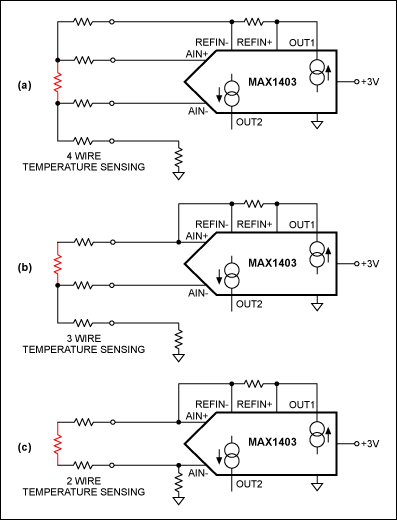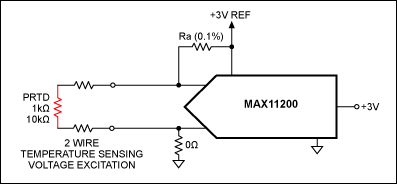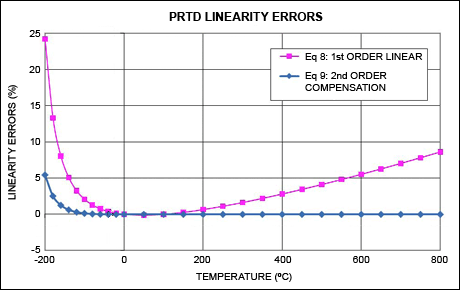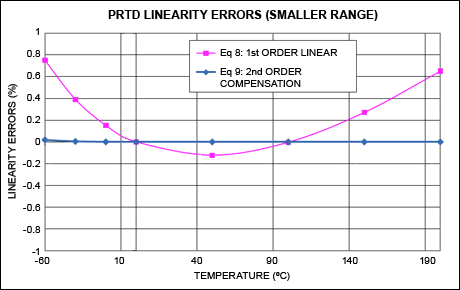# High-Accuracy Temperature Measurements Call for Platinum Resistance Temperature Detectors (PRTDs) and Precision Delta-Sigma ADCs

### Abstract

Advanced industrial and medical applications require temperature measurements with accuracies of ±1°C to ±0.1°C or better, performed with reasonable cost over a wide range of temperature, and often with low power consumption. The temperature ranges common in these applications (-200°C to +1750°C) generally require the use of thermocouples and platinum resistance temperature (RT) detectors, or PRTDs.

A similar version of this article appeared in the August 25, 2011 issue of EDN magazine.

### Introduction

When absolute accuracy and repeatability are critical over temperature ranges of -200°C to +800°C, the best choice for precision industrial and medical applications is the platinum resistance temperature (RT) detector, or PRTD. Platinum is very stable and not affected by corrosion or oxidation. Nickel, copper, and other metals can also be used for RTDs, but those materials are less popular because they are not as stable or repeatable as platinum.
The development of modern PRTD standards such as the European IEC 60751 and the American ASTM 1137 allow substantial interchangeability of sensors between systems, based on the sensors' specified tolerance and temperature coefficients. These standards make it easy to replace a sensor with one from the same or a different manufacturer, while ensuring rated performance with minimal redesign or recalibration of the system.

### PRTD Basics

Three common PRTDs are the PT100, PT500, and PT1000, which exhibit resistance values of 100Ω, 500Ω, and 1000Ω, respectively, at 0°C. Higher-resistance devices such as the PT10000 are available at slightly higher cost. PT100s were more popular historically, but today the trend is toward higher-resistance values that provide higher sensitivity and resolution at little or no extra cost. Typical of these is the PT1000, whose 0°C resistance is 1kΩ.
Manufacturers like Vishay® and JUMO Process Control now offer PRTDs in the standard SMD sizes (very similar to surface-mount resistor packages) with typical prices in the low single-dollar range, depending on the value, size, and tolerance. Such devices reduce the sensor cost substantially and provide designers with the flexibility to place PRTDs on any type of printed circuit board (PCB). The example below includes the popular and cost-effective PTS1206, a 1000Ω PRTD manufactured by Vishay Beyschlag.¹ Traditional methods for PRTD measurement using current-source excitation are shown in Figure 1Figure 1. A PRTD can sense temperature using an interface of four wires (a), three wires (b), or two wires (c). Each design delivers a differential signal to the ADC, here the MAX1403.
For measurements at a distance and with dissimilar lead wires, the 4-wire approach of Figure 1a (Kelvin connection) gives the most accurate results. In this approach the current-carrying wires are separated from the measurement wires; OUT1 provides a 200µA source for the PRTD and OUT2 remains floating in this configuration. For most industrial applications in which the RTD element is not mounted close to the ADC, fewer wires are preferred because each wire adds to the system cost and reliability concerns.
The 3-wire temperature-sensing technique of Figure 1b is more economical and provides accurate readings, if the lead wires are similar. This is why it is the most popular design. The two matched current sources of the MAX1403 ADC cancel the IR errors due to lead wire resistance. OUT1 and OUT2 both source a 200µA current.
The 2-wire technique of Figure 1c is the most economical and is used only when the parasitic wire resistance is known and unchanging. The IR errors of the wires are normally compensated by computation within the microcontroller or DSP. Because the higher resistance in a PT1000 PRTD makes it less sensitive to lead-wire resistance while lowering its self-heating error, it can be connected to the ADC directly, even in a 2-wire configuration.
The MAX11200 ADC is suitable for sampling various types of PRTDs. Some important characteristics of this ADC are listed in Table 1.
Table 1. MAX11200 Key Specifications
 MAX11200 Comments Sample rate (sps) 10 to 120 The MAX11200's variable oversampling rate can be optimized for low noise, and for -150dB line-noise rejection at 50Hz or 60Hz. Channels 1 GPIOs allow external multiplexer control for multichannel measurements. INL (max, ppm) ±10 Provides very good measurement linearity Offset error (µV) ±1 Provides almost zero offset measurements Noise-free resolution (bits) 19.0 at 120sps; 19.5 at 60sps; 21.0 at 10sps Very high dynamic range with low power VDD (V) AVDD (2.7 to 3.6)DVDD (1.7 to 3.6) AVDD and DVDD ranges cover the industry's popular power-supply ranges. ICC (max, µA) 300 Highest resolution-per-unit power in the industry; ideal for portable applications GPIOsAllows external device control, including local multiplexer control. Input range 0 to VREF, ±VREF Wide input ranges Package 16-pin QSOP, 10-pin µMAX® (15mm²) 10-pin µMAX offers very small size for space-constrained designs.

As an alternative to current excitation, you can excite the PRTD with a precision voltage source. Voltage excitation is more desirable for higher-resistance PRTDs, and the same voltage reference that biases the ADC can be used to bias the PRTD. A PRTD can be connected directly to the ADC, and the ADC reference provides PRTD bias current with a single precision resistor (Figure 2). The ADC then measures temperature accurately and ratiometrically.Figure 2. The sensing technique in this circuit is based on voltage excitation, which works best with higher-value PRTDs.
Assuming that the lead wire resistances are orders of magnitude lower than RA and RT, the following formula applies:
 VRTD = VREF × (RT/(RA + RT)) (Eq. 1)
Where RA is the current-limiting resistor; RT is the PRTD resistance at t°C; VRTD is the PRTD voltage; and VREF is the ADC reference voltage. At the same time:
 VRTD = VREF × (AADC/FS) (Eq. 2)
Where AADC is the ADC's output code, and FS is the ADC's full-scale code (i.e., 223-1 for the MAX11200 in a single-ended configuration). Combining Equations 1 and 2:
From Equation 3, it is clear that RA must meet certain precision requirements dictated by the RT specification.

### Error Due to Lead-Wire Resistance

Because the PRTD is a resistive sensor, any resistance introduced by connecting copper extension wires between it and the control instrument adds error, as shown in Figure 3.Figure 3. IR drops in the wires of a 2-wire sensing technique can produce errors at the ADC.
To estimate the errors in a 2-wire circuit, multiply the total length of the extension leads times the resistance per foot for American wire gages (AWG) copper wire, as shown in the Table 2.
Table 2. Wire Gage Resistance Values
 Copper Lead Wire (AWG) Ω/Foot (+25°C) 16 0.0041 18 0.0065 20 0.0103 22 0.0161 24 0.0257 26 0.0418 28 0.0649

As an example, assume that you connected two 3-foot lengths of AWG 22 wire to a PRTD. The lead wire resistance RW is:
 RW = 2 × (3ft.) × (0.0161Ω/ft.) = 0.1Ω (Eq. 4)
The error in temperature reading due to the lead wires is TWER, where TWER = RW/S, and S is the average PRTD sensitivity.
For a PT100 (PTS 1206, 100Ω) device,¹ the average sensitivity is S = 0.385Ω/°C, so:
 TWER = RW/0.385 = 0.26°C (Eq. 5)
For a PT1000 (PTS 1206, 1000Ω) device,¹ the average sensitivity is S = 3.85Ω/°C, so:
 TWER = RW/3.85 = 0.026°C (Eq. 6)
According to the IEC 60751 standard, TWER = 0.026°C for the PT1000 is one order of magnitude below the CLASS F0.3 tolerance of ±0.30°C. This means that a 3-foot, 2-wire configuration can be used directly with the PT1000 without any method of wire compensation. A TWER of 0.26°C for the PT100, however, is comparable with the ±0.30°C tolerance and, therefore, represents an unacceptable level of error for most precision applications. This example demonstrates the advantage of higher-resistance PRTDs in a 2-wire circuit.

### Error Due to PRTD Self-Heating

Another source of error for PRTDs is self-heating of the RTD element itself, as excitation current flows through it. Excitation current flowing through the RTD resistance produces the voltage to be measured. This current should be as high as practical to ensure that the output voltage remains above the ADC's voltage noise level. At the same time, excitation current generates a power loss that warms the temperature sensor, thereby increasing the RTD resistance above the level that it would otherwise assume due to the temperature being measured. This thermal error from RTD power dissipation can be calculated from the package thermal resistance provided in the manufacturer's data sheet. The thermal error from self heating (TTERR in °C) can be calculated using the formula:

 TTERR = IEXT² × RT × KTPACK (Eq. 7)
Where IEXT is the excitation current through the resistive sensing element; RT is the PRTD resistance at the current temperature T°C; and KTPACK is the self-heating error coefficient (0.7°C/mW).¹
In Figure 2, an optimal value of the current-limiting resistor, RA, is determined using Equation 7 for TERR, plus a reference value used in the measurement system (VREF = 3V). Examples of such RA values for the 100Ω PTS 1206 and the 1000Ω PTS 1206 are shown in Table 3.
Table 3. Thermal Error Calculation Budget
 VREF KTPACK T°C RT100 RT1000 RA100 RA1000 TERR100 TERR1000 IEXT100 IEXT1000 VRT100 VRT1000 (V) (C/mW) (°C) (Ω) (Ω) (Ω) (Ω) (°C) (°C) (µA) (µA) (mV) (mV) 3 0.7 -55 78.3 783.2 8200 27000 0.015 0.013 362.4 108.0 28.4 84.6 3 0.7 0 100.0 1000.0 8200 27000 0.019 0.016 361.4 107.1 36.1 107.1 3 0.7 20 107.8 1077.9 8200 27000 0.020 0.018 361.1 106.8 38.9 115.2 3 0.7 155 159.2 1591.9 8200 27000 0.029 0.025 358.9 104.9 57.1 167.0

Using RA = 8.2kΩ for the 100Ω PTS 1206 and RA = 27.0kΩ for the 1000Ω PTS 1206, the maximum thermal error, TERR, is between 0.025°C and 0.029°C in both cases, which is one order of magnitude below the CLASS F0.3 tolerance of ±0.30°C. It is evident that the average excitation currents, IEXT100 and IEXT1000, are very stable and predictable over the temperature ranges indicated in Table 3.
One other conclusion from Table 3 is that the maximum excitation currents are dramatically different from those of the RT100 and RT1000 models: IEXT1000 = 108µA, and IEXT100 = 362.4µA. Thus, an RT1000 is preferable to an RT100 for low-power (portable) instrumentation, because its excitation current is less than one-third of the RT100 current. RA resistors should be the metal-film type with ±0.1% or better tolerances, at least 1/4W power rating, and a low temperature coefficient. To ensure that RA resistors deliver the desired characteristics, they should be acquired from a reputable source.

### Linearity Error of PRTD

PRTDs are nearly linear devices. Depending on the temperature range and other criteria, you can make a linear approximation by calculating the PRTD resistance change over a temperature range of -20°C to +100°C:

 R(t) ≈ R(0)(1 + T × a) (Eq. 8)
R(t) is the PRTD resistance at t°C; R(0) is the PRTD resistance at 0°C; T is the PRTD temperature in °C; and the constant, a, is 0.00385Ω/Ω/°C according to IEC 60751. (In this case a = 0.00385Ω/Ω/°C is actually defined as a mean temperature coefficient between 0°C and 100°C.)¹
PRTD calculations based on Equation 8 are shown in Table 4.
Table 4. PRTD Calculations for the Range -20°C to +100°C
 a Temp RRTD1000 Lin RRTD1000 Nom RA VREF VRTD ADC Code Err (Ω/Ω/°C) (°C) (Ω) (Ω) (Ω) (V) (V) (LSB) (%) 3.85E-03 -20 923.00 921.60 27000 3 0.0991656 277286 0.15 3.85E-03 -10 961.50 960.90 27000 3 0.1031597 288454 0.06 3.85E-03 0 1000.00 1000.00 27000 3 0.1071429 299592 0.00 3.85E-03 10 1038.50 1039.00 27000 3 0.1111151 310699 -0.05 3.85E-03 20 1077.00 1077.90 27000 3 0.1150764 321776 -0.08 3.85E-03 30 1115.50 1116.70 27000 3 0.1190269 332822 -0.11 3.85E-03 40 1154.00 1155.40 27000 3 0.1229665 343838 -0.12 3.85E-03 50 1192.50 1194.00 27000 3 0.1268955 354824 -0.13 3.85E-03 60 1231.00 1232.40 27000 3 0.1308136 365780 -0.11 3.85E-03 100 1385.00 1385.00 27000 3 0.1463801 409308 0.00

In Table 4 the RRTD1000 Lin column represents a linear approximation according to Equation 8. The RRTD1000 Nom column lists the nominal PTS 1206Ω to 1000Ω values according to manufacturing specification EN 60751:2008. The values in the Linearization Error (Err) column for the stated temperature range are all within the range ±0.15%, which is better than the CLASS F0.3 tolerance (±0.30°C) for PTS 1206.
Practical measurements per Table 4 using the MAX11200 ADC (Figure 2) confirm that digital representations of the temperature-reading errors remain within the limits for CLASS F0.3 tolerance. For a wider range and higher accuracy, the temperature-measurement PRTD standard (EN 60751:2008) defines the behavior of platinum resistance vs. temperature by a nonlinear mathematical model called the Callendar-Van Dusen equation.
For temperatures between 0°C to +859°C, the linearization equation requires two coefficients based on the following formula:
 R(t) = R(0)(1 + A × t + B × t²) (Eq. 9)
For temperatures in the range -200°C to 0°C:
 R(t) = R(0)[1 + A × t + B × t² + (t - 100)C × t³] (Eq. 10)
Where R(t) is the PRTD resistance at t°C; R(0) is the PRTD resistance at 0°C; and t is the PRTD temperature in °C. In Equations 9 and 10 A, B, and C are calibration coefficients derived from measurements by RTD manufacturers, as specified by IEC 60751:
A = 3.9083 × 10 - 3°C-1
B = - 5.775 × 10 - 7°C-2
C = - 4.183 × 10 - 12°C-4
The use of Equation 8 shows that nonlinearity errors increase for temperatures outside the band of 0°C to +200°C (Figure 4, pink). Using Equation 9 (blue graph) reduces the error to negligible levels except at very low temperatures.Figure 4. Linearity error vs. temperature for a PRTD, calculated with Equation 8 (pink) and Equation 9 (blue).
Figure 5 enlarges a portion of Figure 4 over a narrower temperature range. It shows that the errors within a smaller range (between -20°C and +100°C), when using Equation 8, are within ±0.15%. These errors become nearly negligible when we use Equation 9. Precision measurements over a wider temperature range (-200°C to +800°C) require the implementation of these linearization algorithms using Equations 9 and 10. (These algorithms will be discussed in a future article.)Figure 5. Amplified view from Figure 4, showing area where the two graphs intersect.

### MAX11200 Measurement Resolution

The MAX11200 is a low-power, 24-bit, delta-sigma ADC suitable for low-power applications that require a wide dynamic range and a high number of noise-free bits. Using this ADC, you can calculate the resolution in temperature for the Figure 2 circuit using Equations 11 and 12:

 RTLSB = (VREF × (TCMAX - TCMIN))/(FS × (VRTMAX - VRTMIN)) (Eq. 11) RTNFR = (VREF × (TCMAX - TCMIN))/(NFR × (VRTMAX - VRTMIN)) (Eq. 12)
Where RTLSB is the PRTD resolution at 1 LSB; RTNFR is the PRTD noise-free resolution (NFR); VREF is the reference voltage; T°CMAX is the maximum measurement temperature; T°CMIN is the minimum measurement temperature; VRTMAX is the PRTD voltage drop at maximum measurement temperature; VRTMIN is the PRTD voltage drop at measurement temperature; FS is the ADC full-scale code for a MAX11200 in a single-ended configuration (223-1); and NFR is the ADC noise-free resolution for a MAX11200 in the single-ended configuration (220-1 at 10sps).
Table 5 lists calculations of the measurement resolution using Equations 11 and 12 for the PTS1206-100Ω and PTS1206-1000Ω.
Table 5. Temperature-Measurement Resolution
 VREF TC RT100 RT1000 RA (100) RA (1000) RTLSB (100) RTLSB (1000) RTNFR (100) RTNFR (1000) (V) (°C) (Ω) (Ω) (Ω) (Ω) (°C/LSB) (°C/LSB) (°C/NFR) (°C/NFR) 3 -55 78.32 783.19 8200 27000 3 0 100 1000 8200 27000 0.00317 0.000926 0.021 0.0073 3 20 107.79 1077.9 8200 27000 3 155 159.19 1591.91 8200 27000

Table 5 provides the calculated values of °C/LSB error and °C/NFR error for a temperature range of -55°C to +155°C. Noise-free resolution (NFR) represents the minimum temperature value that can be differentiated by the ADC. An RTNFR1000 value of 0.007°C/NFR easily allows a temperature resolution better than 0.05°C within the given range, which is more than sufficient for most industrial and medical applications.
Another way to consider the ADC requirement for this application is to look at the expected voltage levels for different temperature points, as shown in Table 6. The last row shows the range of differential voltage output for PRTD100 and PRTD1000 devices. The set of equations on the right calculates how many noise-free codes are produced by the MAX11200 ADC.
Table 6. Temperature Measurement Range for ADC in Figure 6
 TC (°C) VRT (mV) VRT (mV) PRTD100 PRTD1000 -55 28.4 84.6 0 36.1 107.1 20 38.9 115.2 155 57.1 167.0 210 28.75 82.46
Noise free codes = (VMAX - VMIN)/Input referred noise
Noise free codes = 82.46mV/2.86µVP-P
Noise free codes = 28,822 codes
Temp (accy) = 210°C/28.82K
Temp (accy) = 0.007°C

Note that the total range of output signal in PRTD applications is about 82mV. The MAX11200 has an extremely low input-referred noise of 570nV at 10sps, which gives the application a noise-free resolution of 0.007°C over a 210°C span.Figure 6. Block diagram of the precision data-acquisition system (DAS) used for measurements in this article. Based on the MAX11200 ADC (Figure 3), the DAS includes a provision for simple calibration and computer-generated linearization.
As shown in Figure 6, the MAX11200's GPIO1 pin is set as an output to control the relay calibration switch, which selects either the fixed RCAL resistor or the PRTD. This versatility improves the system precision and reduces the required calculations to those for the initial values of RA and RT.

### Conclusion

In recent years the decline in price and package size for a PRTD has made these devices desirable for a variety of precision temperature-sensing applications. Such applications require a low-noise ADC (like the MAX11200) if the ADC and surface-mount PRTD are to be connected directly. Together, the PRTD and ADC provide a temperature-measurement system that is ideal for portable sensing applications. This combination offers high performance, yet is cost effective.
High noise-free resolution, integrated buffers, and GPIO drivers allow the MAX11200 to interface directly with new high-sensitivity PRTDs like the PT1000 without the need for an additional instrumentation amplifier or dedicated current sources. Less wiring and lower thermal errors further reduce the system complexity and cost, allowing the designer to implement a 2-wire interface for distances up to 2 meters.

References
1. PTS Series - Pt-Sensors from Vishay Beyschlag, https://www.vishay.com/docs/28762/ptsserie.pdf.
2. Maxim application note 3775, "Design Considerations for a Low-Cost Sensor and A/D Interface."